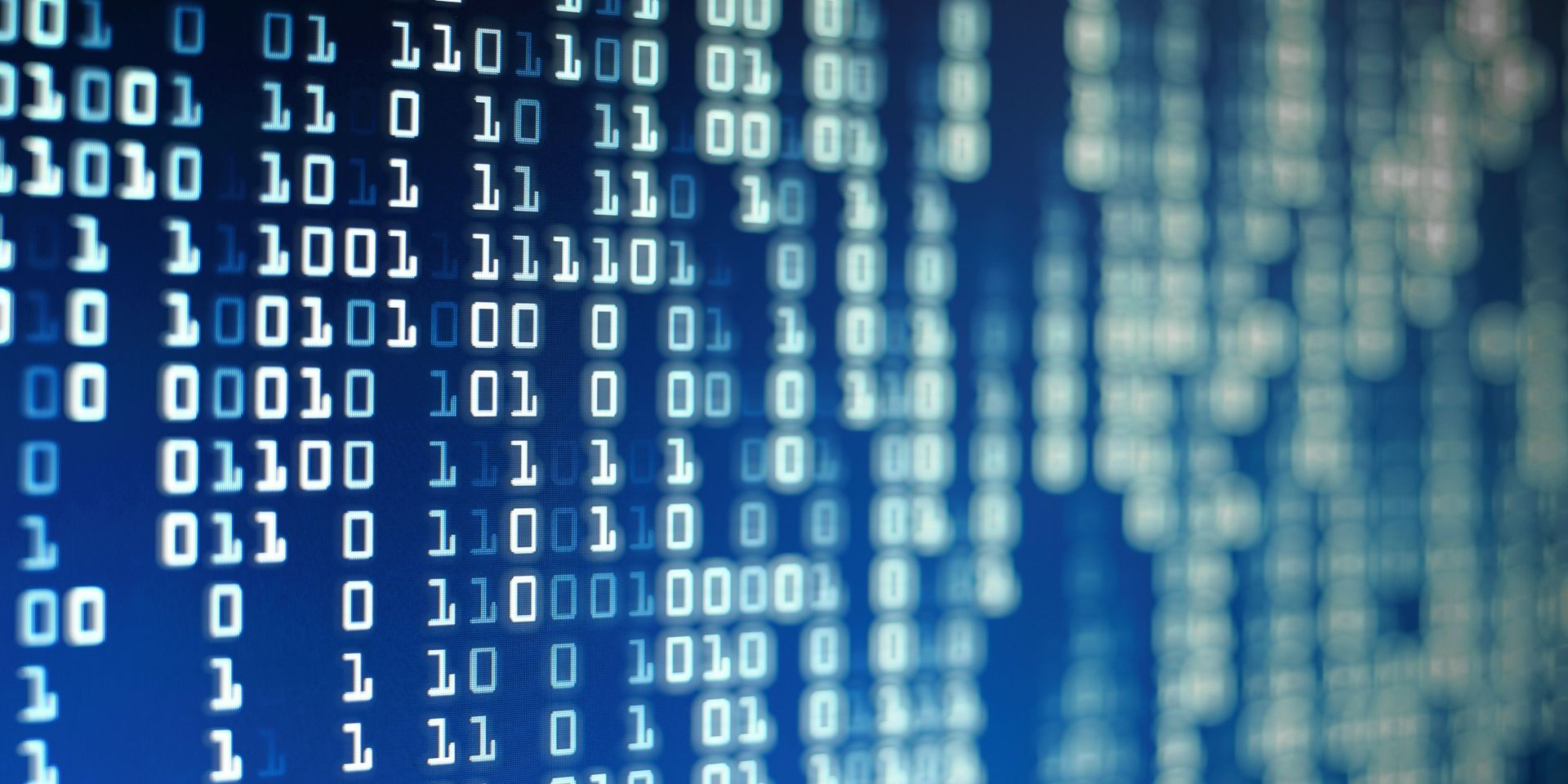Tuition fee €899 one-time

## Overview

Course Description
This course will provide an introduction to data science by covering the basics methods and practices of a data science project. The course is designed around the data science lifecycle to show the techniques for handling a data science project. You will be exposed to basic programming skills in Python and learn how to select, clean, analyse, visualise and interpret data.
By following this course you will have knowledge and insight into: i) differentiating the steps of the data science life cycle; ii) formulating a data research question iii) using Python as the programming language, how data can be analysed; iv) know how basic data analysis algorithms work; v) draw conclusions with regard to the data question/hypothesis by interpreting the data.

This course will make use of lectures and practical assignments organised as follows:
• Lecture: Introduction to data science
• Practical: Introduction to programming in Python: This session will give you the basic skills to program in Python. By the end of this session the student will have gained familiarity with programming and will be able to perform simple data processing in Python.
• Lecture: Introduction to data, data manipulation and visualization
• Practical: Data manipulation and visualization: During this session, the steps of data exploration, selection, cleaning and transformation will be performed via a hands-on assignment.
• Lecture: Introduction to Machine Learning
• Practical Machine Learning: In this session students will be shown hands on examples on how to perform a Logistic Regression and how to use Naive Bayes Classifiers.
• Assessment

Goals
• Get to know the data science lifecycle;
• Use Python as a programming language to perform data analysis tasks;
• Become familiar with the data manipulation process and how to achieve this in Python;
• Get introduced to basic machine learning algorithms and in their application
• Understand data interpretation and visualization tools

Recommended Literature
The course is entirely self-contained. Slides and python notebooks will be provided. To make use of Python Notebooks you will be instructed how to set up python in your device.

Teaching Methods
• Assignments
• Lectures
• Skills
• Work in groups

Assessment Methods
• Assignment and Attendance

Course Coordinator
Dr. Visara Urovi

Application period has ended
Studies commence
12 Jun 2023
Application period has ended
Studies commence
12 Jun 2023